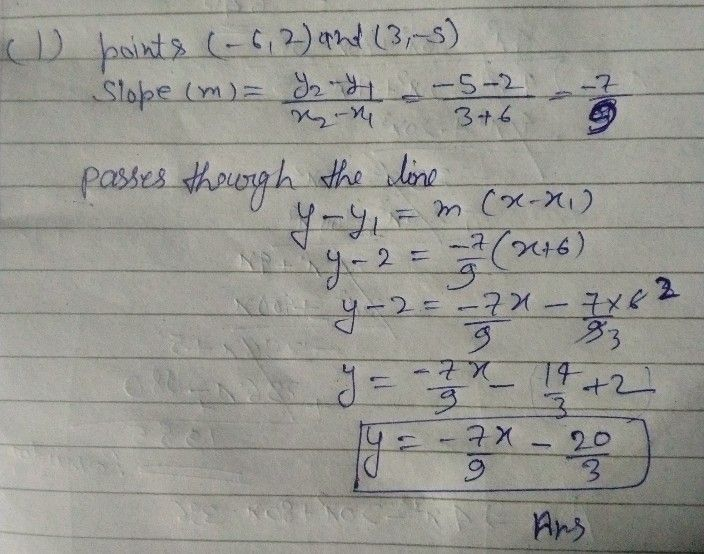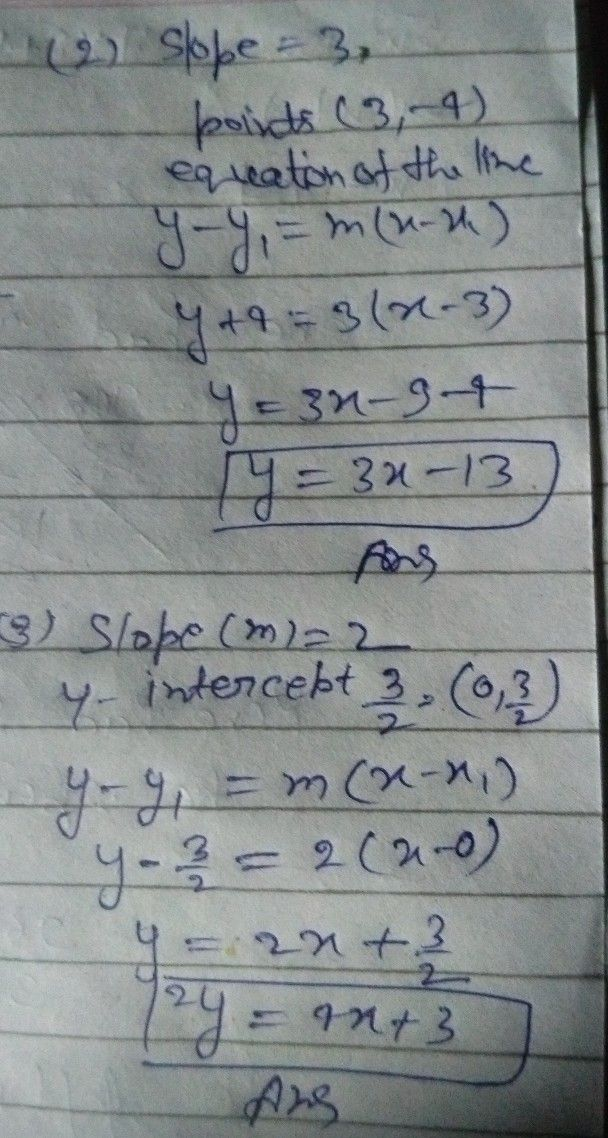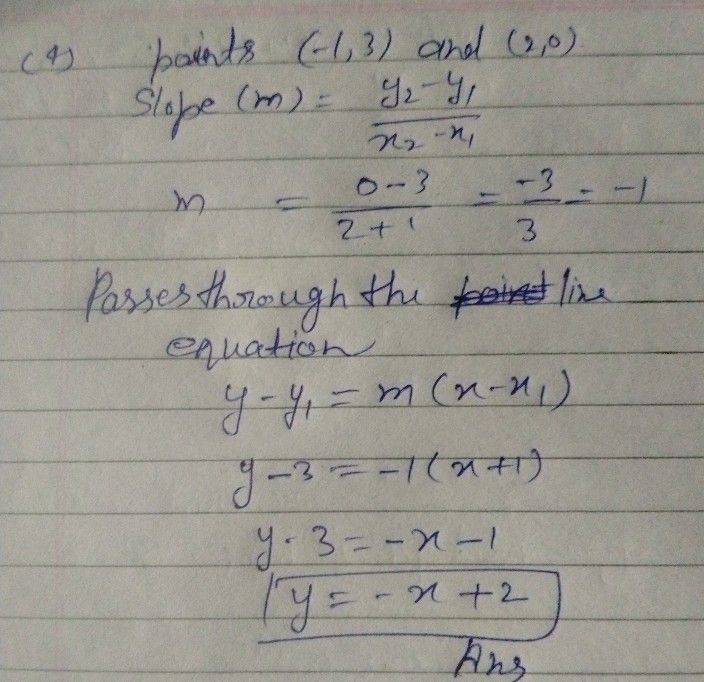Symbol
ProblemActivity $2$ Let's Write an Equation! Directions Write an equation of the tine in slope-untercept fon $y$ The line passes the points $\left(62\right)0$ $35$ $2$ The line passes the point $3-4$ and a slope of 3. $2$ A line that has a slope of $2$ $2$ $0y-=$ $cc9$ of $4$ The line passes through the points (-1,3) and $2$ 0) $6$ The line has a siope -3 and passes through the point